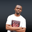Related Tags

javascript
communitycreator

# How to calculate the difference in days between two dates in JSNDUKWE CHIDERA K.

In Javascript, the Date class is used in a variety of ways.

This shot explains how to calculate the number of days between two given dates. This can be achieved in a number of ways, but we will focus on two specific approaches.

### First approach

First, get the internal millisecond value of both dates using the built-in JavaScript getTime() function of the Date class. Convert both dates to milliseconds using the getTime() method of the Date class.

Then, get the difference between both dates and divide the result by one day in a milliseconds value and there you have it.

Let’s see some code snippets.

### Second approach

We can make the calculation outlined in the first approach by creating the present date as an object using the new Date class. This new class, with the use of the get_date() method, provides us with the present date.

Then, we will get another date, for example, Christmas day, by using the Date.getFullYear() method.

We will set an if condition that checks whether we have passed the reference future date, which in this example is Christmas day. If it is already passed, then use the one for next year.

Using the following expression, the result is calculated in milliseconds and then converted into days.

Math.round(christmas() – present_date.getTime())/A_day_in_miliseconds


In this example we calculated the number of days from now until Christmas day.

### Code

RELATED TAGS

javascript
communitycreator

CONTRIBUTORNDUKWE CHIDERA K.
RELATED COURSES

View all Courses

Keep Exploring

Learn in-demand tech skills in half the time